# Expression of a variable from the formula + functions - math problems

#### Number of problems found: 271

• Value of expressionX=2, y=-5 and z=3 what is the value of x-2y?
• Expressions 3If k(x+6)= 4x2 + 20, what is k(10)=?
• Reciprocal value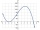How do I calculate a number x that is 9 greater than its reciprocal (1/x)?
• DemographicsThe population grew in the city in 10 years from 30000 to 34000. What is the average annual percentage increase of population?
• Insert 7Insert five harmonic means between 3 and 18
• Tangents to ellipseFind the magnitude of the angle at which the ellipse x2 + 5 y2 = 5 is visible from the point P[5, 1] .
• HP - harmonic progressionDetermine the 8th term of the harmonic progression 2, 4/3, 1,…
• Finding the baseFind the unknown base of percent: 12.5 percent of what = 16?
• Roots and coefficient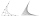In the equation 2x ^ 2 + bx-9 = 0 is one root x1 = -3/2. Determine the second root and the coefficient b.
• DigitsShow that if x, y, z are 3 consecutive nonzero digits, zyx-xyz = 198, where zyx and xyz are three-digit numbers created from x, y, z.
• What isWhat is the annual percentage increase in the city when the population has tripled in 20 years?
• Find the 15Find the tangent line of the ellipse 9 x2 + 16 y2 = 144 that has the slope k = -1
• Together m+wWomen 30%. Men are 360 more. How many are together?
• Price increase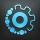A 20 percent price increase meant a 90-crown raise. How many cost a product after?
• The parabolic segment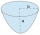The parabolic segment has a base a = 4 cm and a height v = 6 cm. Calculate the volume of the body that results from the rotation of this segment a) around its base b) around its axis.
• Symmetry by plane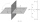Determine the coordinates of a image of point A (3, -4, -6) at a symmetry that is determined by the plane x-y-4z-13 = 0
• Hyperbola equationFind the hyperbola equation with the center of S [0; 0], passing through the points: A [5; 3] B [8; -10]
• Annual growthThe population has grown from 25,000 to 33,600 in 10 years. Calculate what was the average annual population growth in%?
• Geometric progressionIn geometric progression, a1 = 7, q = 5. Find the condition for n to sum first n members is: sn≤217.
• Linear function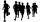Using one of the following forms x+p=q or px=q write an to represent these problems using x as the unknown variable Larry ran 7 more miles than Barry in a month, if Larry ran 20 miles how many did Barry run? please help its due tomorrow

Do you have an interesting mathematical word problem that you can't solve it? Submit a math problem, and we can try to solve it.

We will send a solution to your e-mail address. Solved examples are also published here. Please enter the e-mail correctly and check whether you don't have a full mailbox.

Please do not submit problems from current active competitions such as Mathematical Olympiad, correspondence seminars etc...

Expression of a variable from the formula - math word problems. Functions - math word problems.